Equation of A Circle
Degree Measure of An Arc
More Arcs
Enter Category Name
Mixed Bag
100

A circle has a radius of 4 and center at (−2,5). Write the equation for
this circle.

(x + 2)2 + (y − 5)2 = 16

100

Find the measure of arc DC.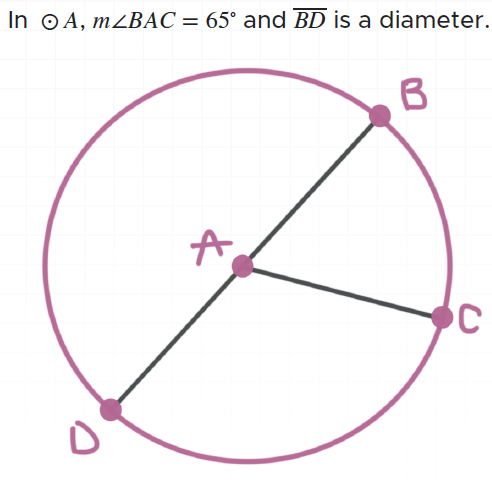115 degrees

100

m∠ABC = 150. Find the measure of arc BC.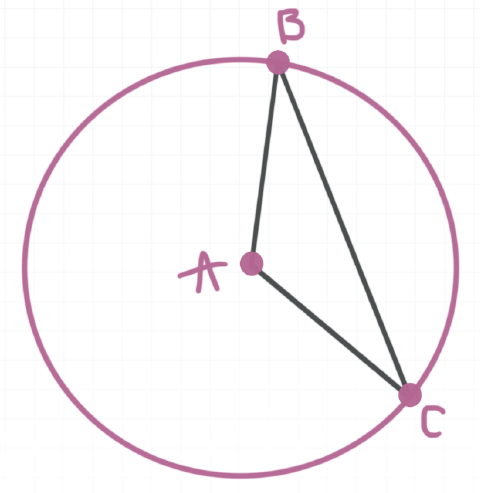150o

200

Find the measure of arc MN.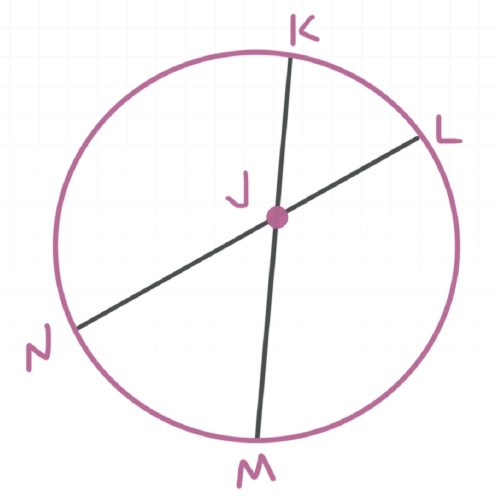54 degrees

200

EG and FH are diameters of ⊙ D. Find the length of arc HG if m∠EDF = 45o and ED = 16. Write the exact value.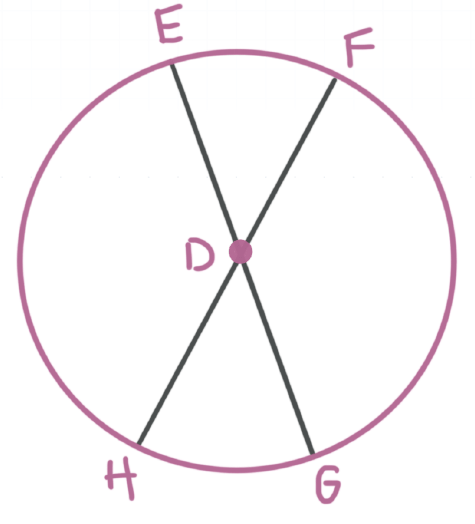4𝝅

300

Find the center and diameter of the circle given by
(x − 3)2 + (y + 2)2 = 9.

Center (3, -2)

Diameter = 6

300

Find the measure of arc FEH.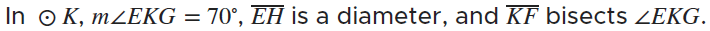215 degrees

300

In ⊙D, m∠ADB = 88∘. Find m∠ACB.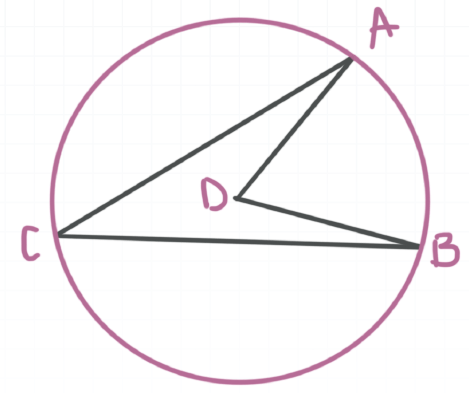44o

400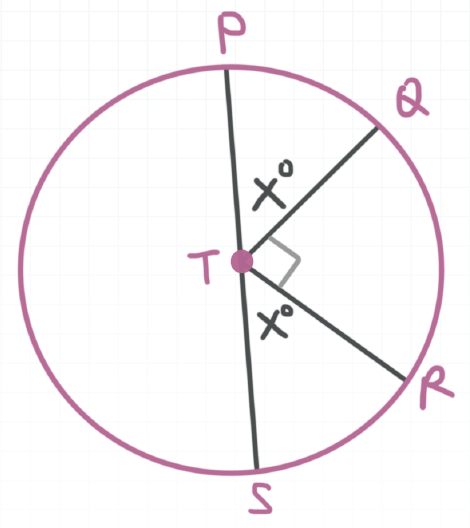45 degrees

400

The area of square ABCD is 144 cm2 and circle P is inscribed in the square. EG and FH are perpendicular to one another, and both are diameters of ⊙P. E, F, G, and H are midpoints of each side of the square.
Find the length of arc EF, rounded to the nearest hundredth.

9.43

500

A circle has a diameter with endpoints at (−3, − 1) and (3,7). Find the equation of the circle.

x2 + (y − 3)2 = 25

500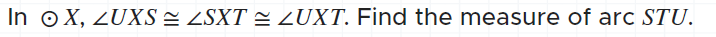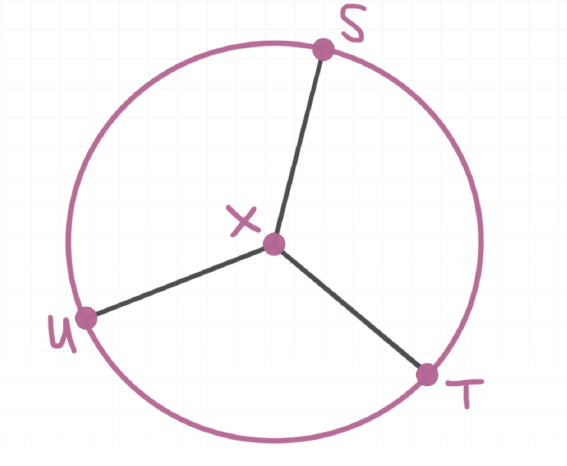240o

500

A cellphone tower services a 17 mile radius. A rest stop on the highway is 6 miles east and 8 miles north of the tower. If you continue to travel due east from the rest stop, for how many more miles will you be in
range of the tower?

9 miles

Click to zoom